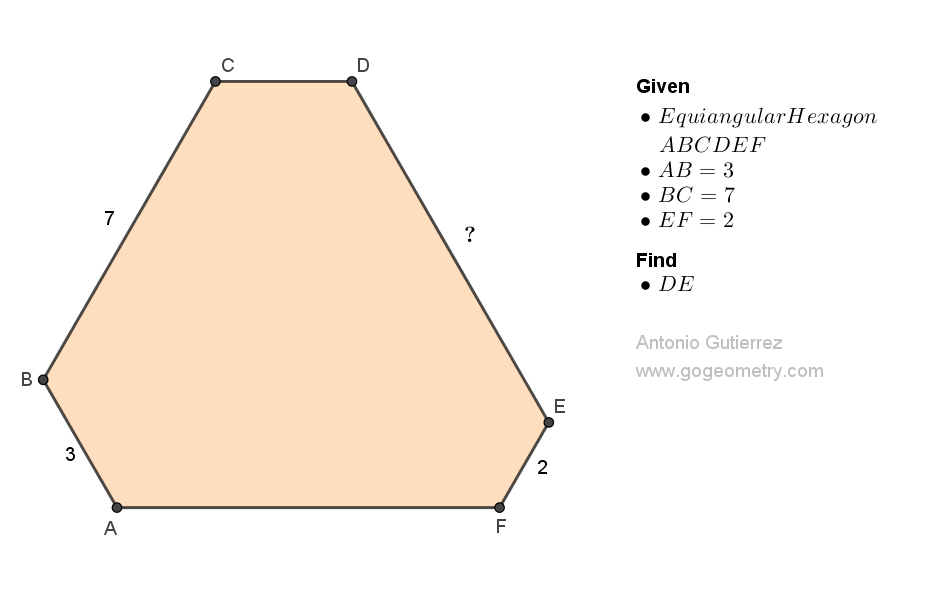# Geometry Problem 1516: Finding the Length of a Side in an Equiangular Hexagon with Given Three Side Lengths. Difficulty Level: High School.

In an equiangular hexagon ABCDEF, AB = 3, BC = 7, and EF = 2. Find the measure of DE.## Definitions and Suggestions

Geometric Shape Theorem
Equiangular Hexagon The sum of the interior angles of an equiangular hexagon is 720 degrees. Each interior angle of an equiangular hexagon measures 120 degrees.
Equilateral Triangle An equilateral triangle is also equiangular, and each of its interior angles measures 60 degrees. All three sides of an equilateral triangle are equal.
Parallel Lines The corresponding angles formed by a transversal intersecting two parallel lines are congruent. The alternate interior angles are congruent.

### Thematic Poem: Finding the Length of a Side in an Equiangular Hexagon

In the realm of shapes and lines,
Geometry holds its design,
A problem to solve with great care,
Equiangular hexagon, quite rare.

With three side lengths at our behest,
We seek the length of the fourth, our quest,
Angles equal, sixty degrees,
Precision paramount, our expertise.

In the world of math and art,
Geometry sets us apart,
Solving puzzles, one by one,
Finding solutions, 'til we're done.

If you're interested in finding more poems with a focus on geometry, you may enjoy this collection: More geometry thematic poems.